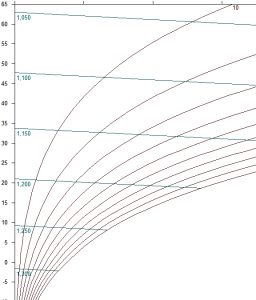# Density of wet air

The density of wet air ρ describes how much a kilogram of substance contains per cubic meter of substance. The density unit ρ therefore has expression in (kg/m3).
Density ρ at 0 °C and 0 m.a.s.l. is for:
dry air: ρ = 1,293 [kg/m3]
water vapor: ρ = 0.804 [kg/m3]

The density ρ depends on three factors:

1. Air pressure: the h-x diagram applies to a certain constant value of air pressure. We must therefore make sure that we use the diagram for the relevant altitude for the calculations. If it is not available, it is necessary to recalculate the relevant state variables.
2. Temperature: The higher the air temperature, the more the air expands and its density decreases.
3. Water vapor content: Water vapor has a lower specific gravity than air. Therefore, with increasing proportion of water vapor, the density of the air-vapor mixture decreases. Therefore, the curves with constant density in the h-x diagram must be inclined to the right.

The graphical representation of wet air densities in the h-x diagram can be as follows: